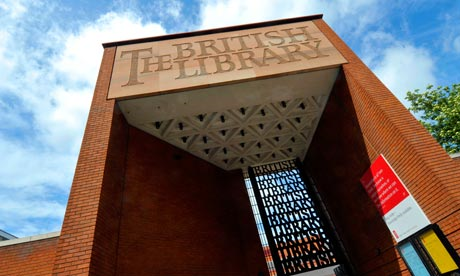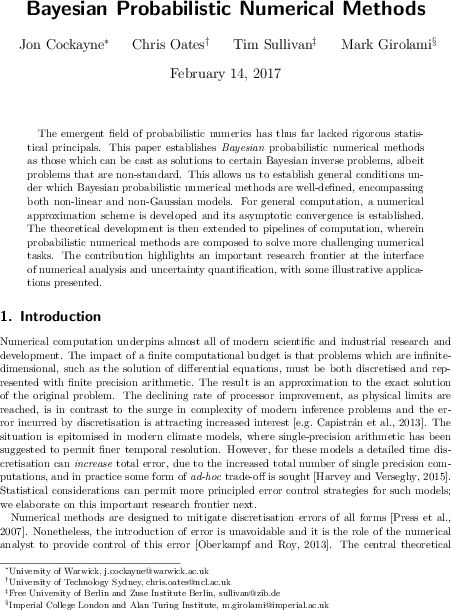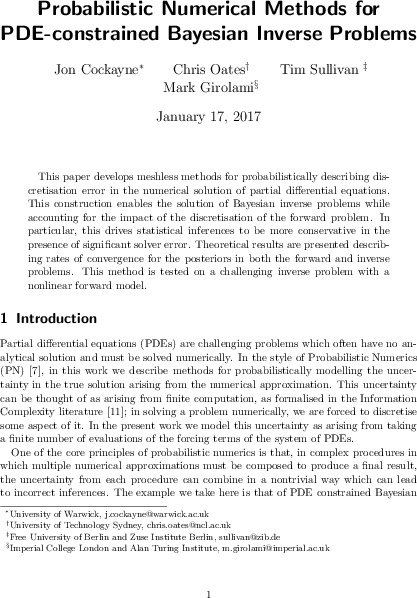Tim Sullivan

#oates

Clear SearchPreprint: Probabilistic numerics retrospective

Chris Oates and I have just uploaded a preprint of our paper “A modern retrospective on probabilistic numerics” to the arXiv.

Abstract. This article attempts to cast the emergence of probabilistic numerics as a mathematical-statistical research field within its historical context and to explore how its gradual development can be related to modern formal treatments and applications. We highlight in particular the parallel contributions of Sul'din and Larkin in the 1960s and how their pioneering early ideas have reached a degree of maturity in the intervening period, mediated by paradigms such as average-case analysis and information-based complexity. We provide a subjective assessment of the state of research in probabilistic numerics and highlight some difficulties to be addressed by future works.

Published on Tuesday 15 January 2019 at 12:00 UTC #preprint #prob-num #oatesPreprint: Optimality of probabilistic numerical methods

Chris Oates, Jon Cockayne, Dennis Prangle, Mark Girolami, and I have just uploaded a preprint of our paper “Optimality criteria for probabilistic numerical methods” to the arXiv.

Abstract. It is well understood that Bayesian decision theory and average case analysis are essentially identical. However, if one is interested in performing uncertainty quantification for a numerical task, it can be argued that the decision-theoretic framework is neither appropriate nor sufficient. To this end, we consider an alternative optimality criterion from Bayesian experimental design and study its implied optimal information in the numerical context. This information is demonstrated to differ, in general, from the information that would be used in an average-case-optimal numerical method. The explicit connection to Bayesian experimental design suggests several distinct regimes in which optimal probabilistic numerical methods can be developed.

Published on Tuesday 15 January 2019 at 11:00 UTC #preprint #prob-num #oates #cockayne #prangle #girolamiProbNum 2018

Next week Chris Oates and I will host the SAMSI–Lloyds–Turing Workshop on Probabilistic Numerical Methods at the Alan Turing Institute, London, housed in the British Library. The workshop is being held as part of the SAMSI Program on Quasi-Monte Carlo and High-Dimensional Sampling Methods for Applied Mathematics.

The accuracy and robustness of numerical predictions that are based on mathematical models depend critically upon the construction of accurate discrete approximations to key quantities of interest. The exact error due to approximation will be unknown to the analyst, but worst-case upper bounds can often be obtained. This workshop aims, instead, to further the development of Probabilistic Numerical Methods, which provide the analyst with a richer, probabilistic quantification of the numerical error in their output, thus providing better tools for reliable statistical inference.

This workshop has been made possible by the generous support of SAMSI, the Alan Turing Institute, and the Lloyd's Register Foundation Data-Centric Engineering Programme.

Published on Friday 6 April 2018 at 07:00 UTC #event #prob-num #turing #samsi #oatesPreprint: Bayesian probabilistic numerical methods

Jon Cockayne, Chris Oates, Mark Girolami and I have just uploaded a preprint of our latest paper, “Bayesian probabilistic numerical methods” to the arXiv. Following on from our earlier work “Probabilistic meshless methods for partial differential equations and Bayesian inverse problems”, our aim is to provide some rigorous theoretical underpinnings for the emerging field of probabilistic numerics, and in particular to define what it means for such a method to be “Bayesian”, by connecting with the established theories of Bayesian inversion and disintegration of measures.

Abstract. The emergent field of probabilistic numerics has thus far lacked rigorous statistical principals. This paper establishes Bayesian probabilistic numerical methods as those which can be cast as solutions to certain Bayesian inverse problems, albeit problems that are non-standard. This allows us to establish general conditions under which Bayesian probabilistic numerical methods are well-defined, encompassing both non-linear and non-Gaussian models. For general computation, a numerical approximation scheme is developed and its asymptotic convergence is established. The theoretical development is then extended to pipelines of computation, wherein probabilistic numerical methods are composed to solve more challenging numerical tasks. The contribution highlights an important research frontier at the interface of numerical analysis and uncertainty quantification, with some illustrative applications presented.

Published on Tuesday 14 February 2017 at 12:00 UTC #publication #preprint #prob-num #bayesian #cockayne #oates #girolamiPreprint: Probabilistic numerical methods for PDE-constrained Bayesian inverse problems

Jon Cockayne, Chris Oates, Mark Girolami and I have just uploaded a preprint of our latest paper, “Probabilistic numerical methods for PDE-constrained Bayesian inverse problems” to the arXiv. This paper is intended to complement our earlier work “Probabilistic meshless methods for partial differential equations and Bayesian inverse problems” and to give a more concise presentation of the main ideas, aimed at a general audience.

Published on Wednesday 18 January 2017 at 12:00 UTC #publication #preprint #prob-num #bayesian #inverse-problems #cockayne #oates #girolami